# 660 My Two-Year Blogiversary

Today is my 2 year blogiversary and my 660th post. Thank you to all my readers and followers. I really appreciate all of you.

What better way is there to celebrate than with a gorgeous cake? Enjoy!

The cake method of finding prime factors makes a beautiful cake for the number 660 because 660 has several prime factors and the largest one, 11, looks like a couple of candles to top it off perfectly.660 has a lot of factors, 24 in fact. 660 is a special number for several reasons:

No number less than 660 has more factors than it does, but 360, 420, 480, 504, 540, 600, and 630 each have just as many.

660 has so many factors that it seems natural for it to between twin primes, 659 and 661.

660 is the hypotenuse of the Pythagorean triple 396-528-660. What is the greatest common factor of those three numbers?

660 is the sum of consecutive prime numbers three different ways. Prime number 101 is in two of those ways:

• 157 + 163 + 167 + 173 = 660
• 101 + 103 + 107 + 109 + 113 + 127 = 660
• 67 + 71 + 73 + 79 + 83 + 89 + 97 + 101 = 660

—————————————————————————————————

• 660 is a composite number.
• Prime factorization: 660 = 2 x 2 x 3 x 5 x 11, which can be written 660 = 2² x 3 x 5 x 11
• The exponents in the prime factorization are 2, 1, 1, and 1. Adding one to each and multiplying we get (2 + 1)(1 + 1)(1 + 1)(1 + 1) = 3 x 2 x 2 x 2 = 24. Therefore 660 has exactly 24 factors.
• Factors of 660: 1, 2, 3, 4, 5, 6, 10, 11, 12, 15, 20, 22, 30, 33, 44, 55, 60, 66, 110, 132, 165, 220, 330, 660
• Factor pairs: 660 = 1 x 660, 2 x 330, 3 x 220, 4 x 165, 5 x 132, 6 x 110, 10 x 66, 11 x 60, 12 x 55, 15 x 44, 20 x 33, or 22 x 30
• Taking the factor pair with the largest square number factor, we get √660 = (√4)(√165) = 2√165 ≈ 25.690465.—————————————————————————————————

# 15 is the Magic Sum of a 3 x 3 Magic Square

15 is a composite number. 15 = 1 x 15 or 3 x 5. Factors of 15: 1, 3, 5, 15. Prime factorization: 15 = 3 x 5.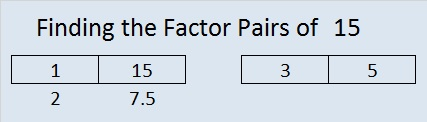When 15 is a clue in the FIND THE FACTORS 1 – 10 or 1 – 12 puzzles, use 3 and 5 as the factors.

If you added the first nine counting numbers together, what sum would you get? What is 1 + 2 +3 + 4+ 5 + 6 + 7 + 8 + 9?

Would you get the same answer by adding (1 + 9) + (2 + 8) + (3 +7) + (4 + 6) + 5?

These are two of the many fun questions you can explore when you try to make a magic square. What is a magic square? If you can place the numbers from 1 to 9 in the box below so that the sum of any row, column, or diagonal will equal the sum of any other row, column, or diagonal, then you will have made a 3 x 3 magic square. The sum of a row, column, or diagonal in a magic square is called the magic sum.Clearly it is not a magic square yet. In fact, only one of the numbers is positioned where it needs to be. Which number do you think is already in the correct position?

When it becomes a magic square, what will the magic sum be? One student noticed that in its current state the sums of the rows are 6, 15, and 24. The sums of the columns are 12, 15, 18. The sums of the diagonals are 15 and 15. Since 15 occurs most often, could the magic sum be 15? One way to determine what the magic sum should be is to add the sums of all three rows and then divide by the number of rows. Since 1 + 2 + 3 + 4 + 5 + 6 + 7 + 8 + 9 = 45 and 45 ÷ 3 = 15, then 15 is indeed the magic sum.

Here are a few easy-to-remember steps to construct a 3 x 3 magic square quickly.

Step 1: Draw a tic-tac-toe board and put 5 in the middle.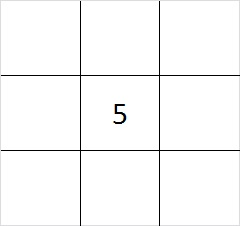Step 2: Put one of the even numbers in one of the corners.  You have four different choices, 2, 4, 6, or 8. The illustration is for the number 2, but any of the even numbers will work.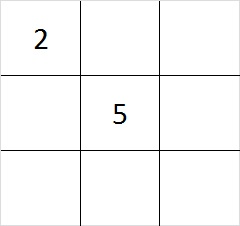Step 3: Subtract your even number from 10 to find its partner. 4 + 6 are partners and so are 2 + 8. Put the partner of the number you chose for step 1 in the corner that is diagonal to it.Step 4: Put the other two even numbers in the remaining corners. Yes, you have two choices where to put the numbers. Either choice will work.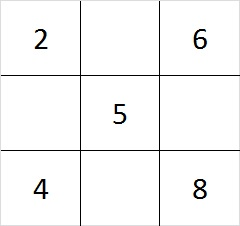Step 5: Since 6 + 8 = 14 and 15 – 14 = 1, put 1 in the cell between the 6 and the 8. Do similar addition and subtraction problems on each side of the square to determine where to place the 3, 7, and 9. You can work clockwise or counter clockwise, or skip around the square doing the addition and subtraction problems; it doesn’t matter.

This finished magic square looks like this: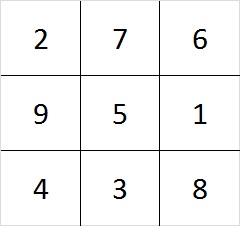Check it out! Every row, column, and diagonal adds up to 15!

As we created the square, we made choices. First we chose between 4 even numbers, and later we had 2 more choices. Notice that 4 x 2 = 8. There are 8 different ways to make a 3 x 3 magic square! (However, they are all really the same square turned upside down, rolled on its side, viewed from the back. etc.)

There are 880 different ways to make a 4 x 4 magic square. Look over the related articles at the end of this post to learn more about magic squares that are bigger than 3 x 3.

Speaking of magic squares, when I look at the square logic puzzle below, something magical happens. This puzzle has nine clues in it, and all of them are perfect squares. I can use those nine clues to construct a complete multiplication table. If you finish the same puzzle, your multiplication table will look exactly like mine because this puzzle has only one solution.The level 3 puzzle below is only a little bit more difficult. To solve it place the numbers 1 – 10 in the top row and again in the first column so that those placed numbers are the factors of the given clues. Again there is only one solution, and you will need to use logic to find it. Click 10 Factors 2014-01-06 for more puzzles and last week’s answers.May we all find a little bit more magic in our lives!